# Excel formula to get day of week from date

In this article, we'll explore different methods to extract days of the week from calendar date in Excel such as formulas and custom number formats.

When you're using Excel to manage project deadlines, schedule appointments, or analyze data trends, it's often handy to know the day names for specific dates. Excel has a variety of tools to help you figure this out. In this article, we'll show you some straightforward ways to get the day of the week from a date, whether you want it as word or number.

## Get day of week from date using TEXT formula

One of the simplest ways to find the day names from a date in Excel is by using the TEXT function. Here’s how:

1. Let's assume that the original date is in cell A3.
2. In another cell (let's say B3), enter one of these formulas:

To return the full name of the day such as "Monday" or "Tuesday", the formula is:

`=TEXT(A3, "dddd")`

To get a shorter version of the day names like "Mon" or "Tue", use this day of week code:

`=TEXT(A3, "ddd")`

3. Press Enter, and cell B3 will display the day name corresponding to the date in cell A3.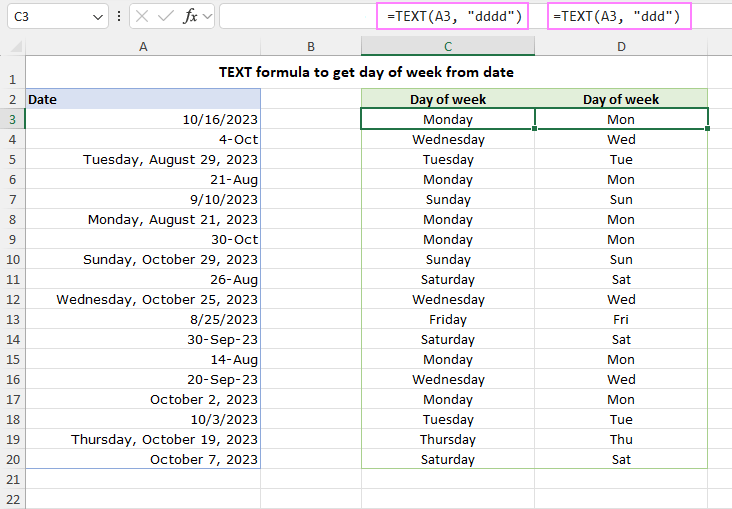It's important to note that the TEXT function always gives you the result as text, regardless of the original cell's format. If you plan to use this output for further calculations, consider using a custom format instead of a formula.

## Find day names with WEEKDAY formula

Another simple formula for days of the week in Excel is WEEKDAY.

By default, the WEEKDAY function returns a number between 1 and 7. In this system, Sunday is considered as 1, Monday as 2, and so on, but you can change this by specifying a different return_type argument.

### Convert day of week to number

If your goal is to get the day of the week number, then the WEEKDAY function in its basic form is all you need:

`=WEEKDAY(A1)`

If cell A1 contains a date like "18-Sep-2023" (Monday), the formula will return 2; or 3 if A1 contains "19-Sep-2023" (Tuesday).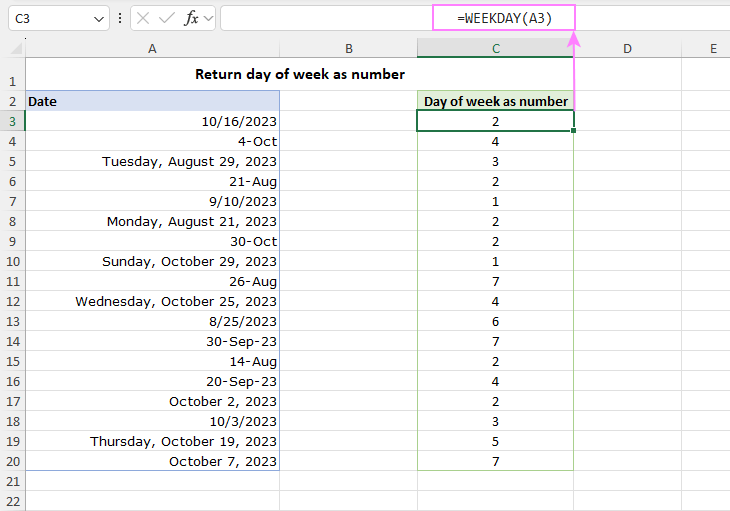### Convert calendar date to day of week

If you prefer having the day names, not numbers, you can combine the WEEKDAY function with the TEXT function.

For example, to convert a calendar date in cell A1 to the day of week, use one of these formulas:

`=TEXT(WEEKDAY(A1), "dddd")`

Or

`=TEXT(WEEKDAY(A1), "ddd")`

The difference is that the first formula returns the full name of the day while the second formula displays a shorter version of the name.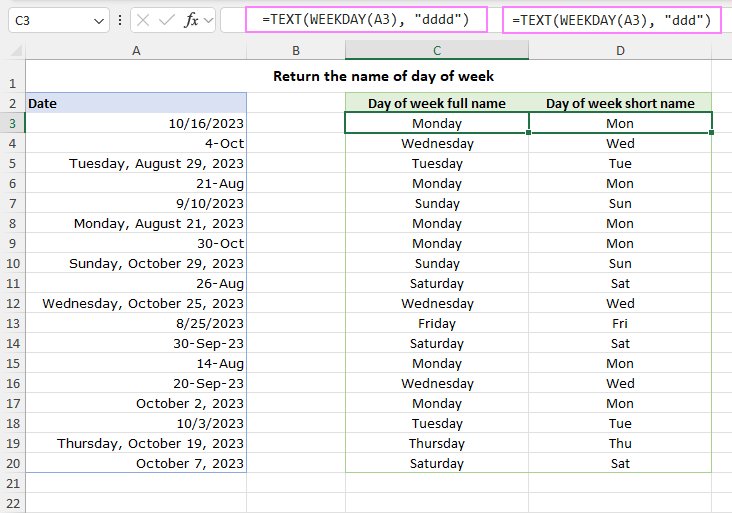## Return day name in any format using CHOOSE formula

If you want to create your custom labels or notations for the day names, you can do this by using WEEKDAY in combination with the CHOOSE function. Here's how it works:

• CHOOSE returns a value from a predefined list based on an index number.
• WEEKDAY calculates the index number corresponding to the day of the week for a given date.

In other words, this formula maps the numeric output of the WEEKDAY function to the corresponding name that you've hardcoded into the CHOOSE function.

Let's assume that your date is in cell A3, and you want to show the day names as 2-letter abbreviations such as "Su" for Sunday, "Mo" for Monday, etc. The following formula works a treat:

`=CHOOSE(WEEKDAY(A3), "Su","Mo","Tu","We","Th","Fr","Sa")`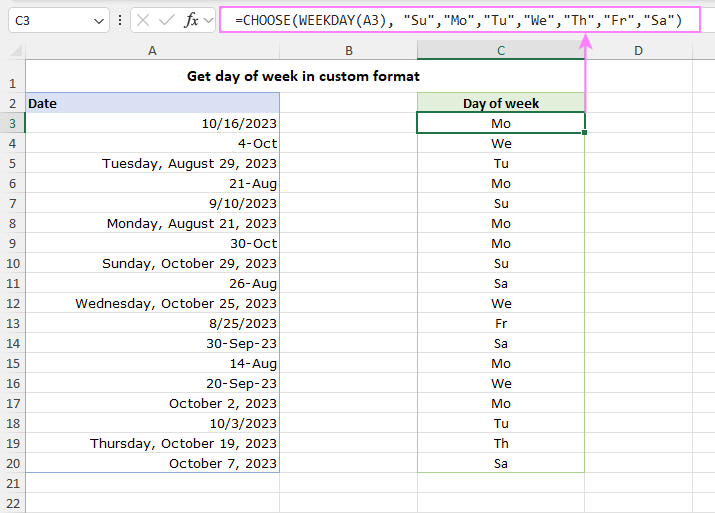## Display day of week from date using custom format

Another way to show the day names in Excel is by applying a custom number format. This approach allows you to keep the original date value in the cell while visually displaying the day of the week. Here's how to do it:

1. Select the cell(s) containing the date you want to format.
2. Right-click the selected cells to bring up a context menu, then choose Format Cells.
3. In the Format Cells dialog box, navigate to the Number tab and select Custom in the Category list.
4. In the Type field, enter the desired code for the day of week:
• dddd – the code for the full day names (e.g. Sunday).
• ddd – the code for abbreviated day names (e.g. Sun).
5. Click OK to apply the custom format.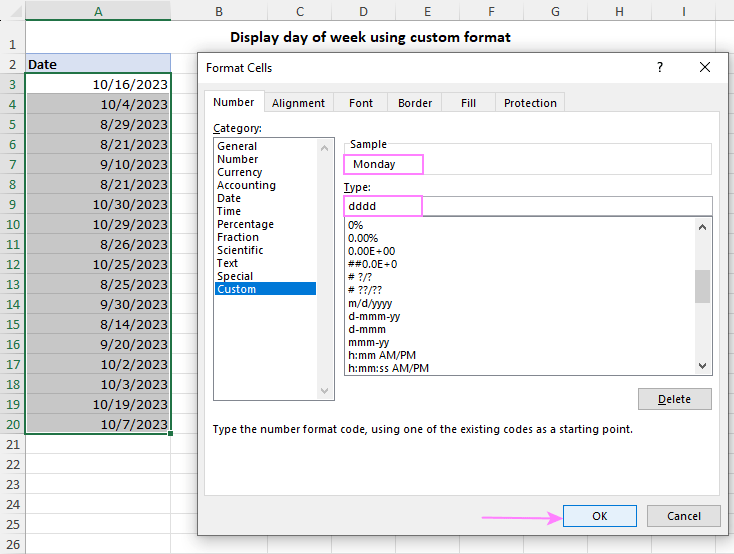Unlike Excel formulas, this method does not change the underlying values of the cells, but only changes how they appear in cells.

In the screenshot below, you can see three columns with the same dates. Column A displays the dates in the default date format, column B shows full day names (“dddd” format), and column C shows abbreviated names (“ddd” format). If you check the formula bar, you'll notice that all three columns retain the original date values. This means you can use these dates in any calculations as fully functional Excel dates while providing a desired visual representation of the day of the week.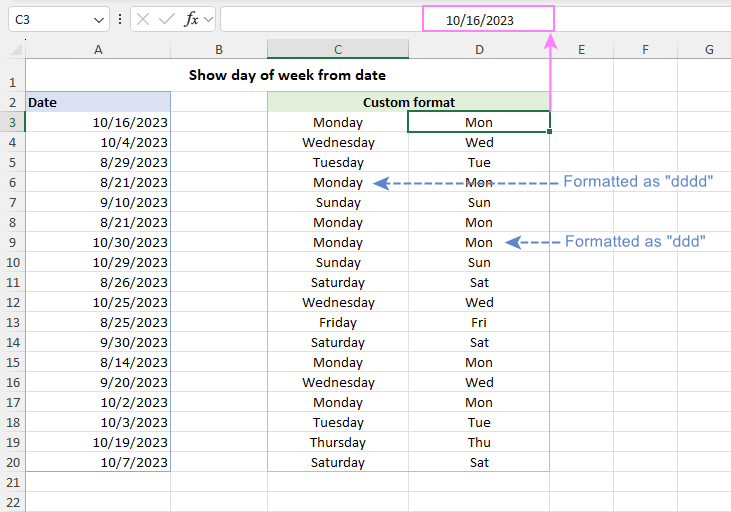These are some of the ways to get the day of week from date in Excel. You can choose any method that suits your needs and preferences. Thanks for reading, and we'll be back with more helpful tips soon!